Case Based Questions Test: Polynomials - 2

# Case Based Questions Test: Polynomials - 2 - Class 10

Test Description

## 10 Questions MCQ Test Mathematics (Maths) Class 10 - Case Based Questions Test: Polynomials - 2

Case Based Questions Test: Polynomials - 2 for Class 10 2023 is part of Mathematics (Maths) Class 10 preparation. The Case Based Questions Test: Polynomials - 2 questions and answers have been prepared according to the Class 10 exam syllabus.The Case Based Questions Test: Polynomials - 2 MCQs are made for Class 10 2023 Exam. Find important definitions, questions, notes, meanings, examples, exercises, MCQs and online tests for Case Based Questions Test: Polynomials - 2 below.
Solutions of Case Based Questions Test: Polynomials - 2 questions in English are available as part of our Mathematics (Maths) Class 10 for Class 10 & Case Based Questions Test: Polynomials - 2 solutions in Hindi for Mathematics (Maths) Class 10 course. Download more important topics, notes, lectures and mock test series for Class 10 Exam by signing up for free. Attempt Case Based Questions Test: Polynomials - 2 | 10 questions in 20 minutes | Mock test for Class 10 preparation | Free important questions MCQ to study Mathematics (Maths) Class 10 for Class 10 Exam | Download free PDF with solutions
 1 Crore+ students have signed up on EduRev. Have you?
Case Based Questions Test: Polynomials - 2 - Question 1

### Direction: Read the following text and answer the following questions on the basis of the same:An asana is a body posture, originally and still a general term for a sitting meditation pose, and later extended in hatha yoga and modern yoga as exercise, to any type of pose or position, adding reclining, standing, inverted, twisting, and balancing poses. In the figure, one can observe that poses can be related to representation of quadratic polynomial.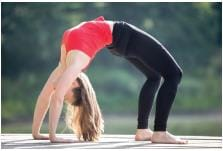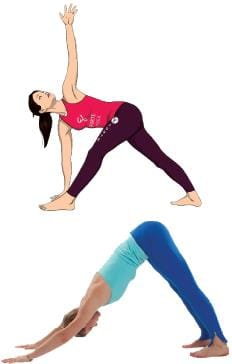The zeroes of the quadratic polynomial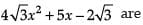Detailed Solution for Case Based Questions Test: Polynomials - 2 - Question 1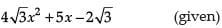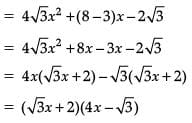Hence, zeroes of polynomial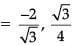Case Based Questions Test: Polynomials - 2 - Question 2

### Direction: Read the following text and answer the following questions on the basis of the same:An asana is a body posture, originally and still a general term for a sitting meditation pose, and later extended in hatha yoga and modern yoga as exercise, to any type of pose or position, adding reclining, standing, inverted, twisting, and balancing poses. In the figure, one can observe that poses can be related to representation of quadratic polynomial.The graph of parabola opens downwards, if _______

Detailed Solution for Case Based Questions Test: Polynomials - 2 - Question 2
If a > 0 (positive) then the parabola opens upward. If a < 0 (negative) then the parabola opens downward.
Case Based Questions Test: Polynomials - 2 - Question 3

### Direction: Read the following text and answer the following questions on the basis of the same:An asana is a body posture, originally and still a general term for a sitting meditation pose, and later extended in hatha yoga and modern yoga as exercise, to any type of pose or position, adding reclining, standing, inverted, twisting, and balancing poses. In the figure, one can observe that poses can be related to representation of quadratic polynomial.The two zeroes in the above shown graph are

Detailed Solution for Case Based Questions Test: Polynomials - 2 - Question 3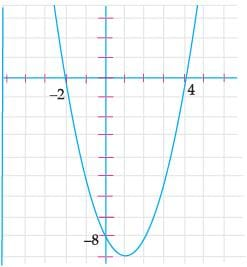Here,

Zeroes are the values of x where graph intersects the x-axis

Therefore, Zeroes are -2 and 4

Case Based Questions Test: Polynomials - 2 - Question 4

Direction: Read the following text and answer the following questions on the basis of the same:

An asana is a body posture, originally and still a general term for a sitting meditation pose, and later extended in hatha yoga and modern yoga as exercise, to any type of pose or position, adding reclining, standing, inverted, twisting, and balancing poses. In the figure, one can observe that poses can be related to representation of quadratic polynomial.The shape of the poses shown is

Detailed Solution for Case Based Questions Test: Polynomials - 2 - Question 4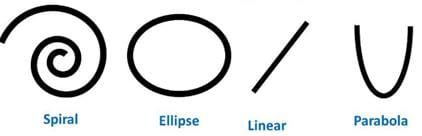The shape of the poses shown is Parabola.

Case Based Questions Test: Polynomials - 2 - Question 5

Direction: Read the following text and answer the following questions on the basis of the same:

An asana is a body posture, originally and still a general term for a sitting meditation pose, and later extended in hatha yoga and modern yoga as exercise, to any type of pose or position, adding reclining, standing, inverted, twisting, and balancing poses. In the figure, one can observe that poses can be related to representation of quadratic polynomial.In the graph, how many zeroes are there for the polynomial?Detailed Solution for Case Based Questions Test: Polynomials - 2 - Question 5
Number of zeroes is equal to number of times parabola intersects the x-axis

Therefore, Number of zeroes = 2

Case Based Questions Test: Polynomials - 2 - Question 6

Direction: Read the following text and answer the following questions on the basis of the same:

Applications of Parabolas: Highway Overpasses/ Underpasses

A highway underpass is parabolic in shape.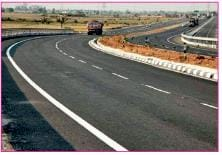Shape of Cross Slope: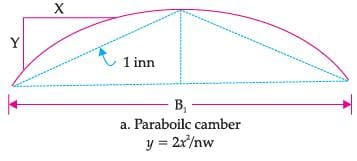Parabola

A parabola is the graph that results from p(x) = ax2 + bx + c.

Parabolas are symmetric about a vertical line known as the Axis of Symmetry.

The Axis of Symmetry runs through the maximum or minimum point of the parabola which is called the vertex.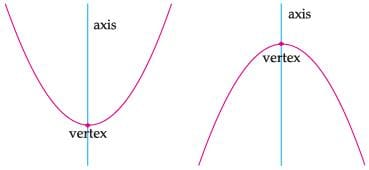The number of zeroes that polynomial f(x) = (x – 2)2 + 4 can have is:

Detailed Solution for Case Based Questions Test: Polynomials - 2 - Question 6
We have,

f(x) = (x – 2)2 + 4

= x2 + 4 – 4x + 4

= x2 – 4x + 8.

i.e., It has no factorisation.

Hence no real value of x is possible, i.e., no zero.

Case Based Questions Test: Polynomials - 2 - Question 7

Direction: Read the following text and answer the following questions on the basis of the same:

Applications of Parabolas: Highway Overpasses/ Underpasses

A highway underpass is parabolic in shape.Shape of Cross Slope:Parabola

A parabola is the graph that results from p(x) = ax2 + bx + c.

Parabolas are symmetric about a vertical line known as the Axis of Symmetry.

The Axis of Symmetry runs through the maximum or minimum point of the parabola which is called the vertex.The highway overpass is represented graphically. Zeroes of a polynomial can be expressed graphically. Number of zeroes of polynomial is equal to number of points where the graph of polynomial:

Detailed Solution for Case Based Questions Test: Polynomials - 2 - Question 7
We know that the number of zeroes of polynomial is equal to number of points where the graph of polynomial intersects X-axis.
Case Based Questions Test: Polynomials - 2 - Question 8

Direction: Read the following text and answer the following questions on the basis of the same:

Applications of Parabolas: Highway Overpasses/ Underpasses

A highway underpass is parabolic in shape.Shape of Cross Slope:Parabola

A parabola is the graph that results from p(x) = ax2 + bx + c.

Parabolas are symmetric about a vertical line known as the Axis of Symmetry.

The Axis of Symmetry runs through the maximum or minimum point of the parabola which is called the vertex.The representation of Highway Underpass whose one zero is 6 and sum of the zeroes is 0, is:

Detailed Solution for Case Based Questions Test: Polynomials - 2 - Question 8
x2 – 36 = 0

⇒ x2 = 36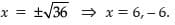Case Based Questions Test: Polynomials - 2 - Question 9

Direction: Read the following text and answer the following questions on the basis of the same:

Applications of Parabolas: Highway Overpasses/ Underpasses

A highway underpass is parabolic in shape.Shape of Cross Slope:Parabola

A parabola is the graph that results from p(x) = ax2 + bx + c.

Parabolas are symmetric about a vertical line known as the Axis of Symmetry.

The Axis of Symmetry runs through the maximum or minimum point of the parabola which is called the vertex.If the highway overpass is represented by x2 – 2x – 8.Then its zeroes are

Detailed Solution for Case Based Questions Test: Polynomials - 2 - Question 9
x2 – 2x – 8 = 0

or, x2 – 4x + 2x – 8 = 0

or, x(x – 4) + 2(x – 4) = 0

or, (x – 4) (x + 2) = 0

or, x = 4, x = – 2

Case Based Questions Test: Polynomials - 2 - Question 10

Direction: Read the following text and answer the following questions on the basis of the same:

Applications of Parabolas: Highway Overpasses/ Underpasses

A highway underpass is parabolic in shape.Shape of Cross Slope:Parabola

A parabola is the graph that results from p(x) = ax2 + bx + c.

Parabolas are symmetric about a vertical line known as the Axis of Symmetry.

The Axis of Symmetry runs through the maximum or minimum point of the parabola which is called the vertex.Graph of a quadratic polynomial is a:

Detailed Solution for Case Based Questions Test: Polynomials - 2 - Question 10
Here, the given graph of a quadratic polynomial is a parabola.

## Mathematics (Maths) Class 10

115 videos|478 docs|129 tests
Information about Case Based Questions Test: Polynomials - 2 Page
In this test you can find the Exam questions for Case Based Questions Test: Polynomials - 2 solved & explained in the simplest way possible. Besides giving Questions and answers for Case Based Questions Test: Polynomials - 2, EduRev gives you an ample number of Online tests for practice

## Mathematics (Maths) Class 10

115 videos|478 docs|129 tests